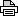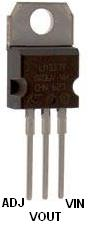REUK.CO.UK
The Renewable Energy Website

This is the printer friendly version of the LM317 Voltage Calculator article from the Renewable Energy Website REUK.co.uk.

Click here to printthis article.

Printed at 03:31am 14th August 2020

# LM317 Voltage Calculator

## A handy online output voltage calculator for the LM317, LM338, and other linear voltage regulators

The LM317 (1) (LM317T), and high current LM338 (2) (LM338T) are voltage regulators which can take an input voltage of 3-40 Volts DC, and output a fixed output voltage from 1.2 to 37 Volts DC.The output voltage from the LM317 and LM338 is set using two resistors (3) (R1 and R2) with their values chosen according to the following equation:

 VOUT = 1.25 * ( 1 + R2/R1 )

# REUK LM317/LM338 Voltage Calculator

Below is our automated voltage calculator for the LM317 and LM338 regulators. Simply enter your target output voltage Vout and chosen value for R1 (100 to 1000 Ohms), click Calculate, and the required value of R2 will be displayed.

 Vout: Target Output Voltage1.5 to 37.0 Volts R1: R1 Resistor Value100-1000 Ohms R2: Click Calculate to display thecalculated R2 value here.
The results given above can be used to put together a voltage regulator, but the calculated value of R2 will not be a standard/stock resistor value. Since the accuracy of the voltage regulator itself will be up to 5% out, and resistor values are not exact, it is common to use a Potentiometer (4) (aka variable resistor or trim pot) for some or all of R2. This enables the output voltage to be manually fine-tuned to provide the exact voltage required.
Enter values of R1 and R2 below to calculate the corresponding value of Vout.

 R1: R1 Resistor Value100-1000 Ohms R2: R2 Resistor Value Vout: Click Calculate to display thecalculated Vout value here.

# Output Voltage Tables for Stock R1 and R2 Values

Where a very accurate voltage is not required, it is much easier to use standard (stock) resistors to put together an LM317 or LM338 voltage regulator. Below are tables of output voltages for different combinations of the most commonly found resistors.

## R1 vs R2 Grid

 R2\R1 150 180 220 240 270 330 370 390 470 68 1.82 1.72 1.64 1.6 1.56 1.51 1.48 1.47 1.43 82 1.93 1.82 1.72 1.68 1.63 1.56 1.53 1.51 1.47 100 2.08 1.94 1.82 1.77 1.71 1.63 1.59 1.57 1.52 120 2.25 2.08 1.93 1.88 1.81 1.7 1.66 1.63 1.57 150 2.5 2.29 2.1 2.03 1.94 1.82 1.76 1.73 1.65 180 2.75 2.5 2.27 2.19 2.08 1.93 1.86 1.83 1.73 220 3.08 2.78 2.5 2.4 2.27 2.08 1.99 1.96 1.84 240 3.25 2.92 2.61 2.5 2.36 2.16 2.06 2.02 1.89 270 3.5 3.13 2.78 2.66 2.5 2.27 2.16 2.12 1.97 330 4 3.54 3.13 2.97 2.78 2.5 2.36 2.31 2.13 370 4.33 3.82 3.35 3.18 2.96 2.65 2.5 2.44 2.23 390 4.5 3.96 3.47 3.28 3.06 2.73 2.57 2.5 2.29 470 5.17 4.51 3.92 3.7 3.43 3.03 2.84 2.76 2.5 560 5.92 5.14 4.43 4.17 3.84 3.37 3.14 3.04 2.74 680 6.92 5.97 5.11 4.79 4.4 3.83 3.55 3.43 3.06 820 8.08 6.94 5.91 5.52 5.05 4.36 4.02 3.88 3.43 1000 9.58 8.19 6.93 6.46 5.88 5.04 4.63 4.46 3.91 1200 11.25 9.58 8.07 7.5 6.81 5.8 5.3 5.1 4.44 1500 13.75 11.67 9.77 9.06 8.19 6.93 6.32 6.06 5.24 1800 16.25 13.75 11.48 10.63 9.58 8.07 7.33 7.02 6.04 2200 19.58 16.53 13.75 12.71 11.44 9.58 8.68 8.3 7.1 2700 23.75 20 16.59 15.31 13.75 11.48 10.37 9.9 8.43 3300 28.75 24.17 20 18.44 16.53 13.75 12.4 11.83 10.03

## Sorted List of Output Voltages with R1 and R2

 1.43V R1 = 470, R2 = 681.47V R1 = 470, R2 = 821.48V R1 = 370, R2 = 681.51V R1 = 330, R2 = 681.51V R1 = 390, R2 = 821.52V R1 = 470, R2 = 1001.53V R1 = 370, R2 = 821.56V R1 = 330, R2 = 821.57V R1 = 270, R2 = 681.57V R1 = 470, R2 = 1201.57V R1 = 390, R2 = 1001.59V R1 = 370, R2 = 1001.60V R1 = 240, R2 = 681.63V R1 = 330, R2 = 1001.63V R1 = 270, R2 = 821.64V R1 = 390, R2 = 1201.64V R1 = 220, R2 = 681.65V R1 = 470, R2 = 1501.66V R1 = 370, R2 = 1201.68V R1 = 240, R2 = 821.71V R1 = 330, R2 = 1201.71V R1 = 270, R2 = 1001.72V R1 = 220, R2 = 821.72V R1 = 180, R2 = 681.73V R1 = 470, R2 = 1801.73V R1 = 390, R2 = 1501.76V R1 = 370, R2 = 1501.77V R1 = 240, R2 = 1001.81V R1 = 270, R2 = 1201.82V R1 = 150, R2 = 681.82V R1 = 330, R2 = 1501.82V R1 = 180, R2 = 821.83V R1 = 390, R2 = 1801.84V R1 = 470, R2 = 2201.86V R1 = 370, R2 = 1801.88V R1 = 240, R2 = 1201.89V R1 = 470, R2 = 2401.93V R1 = 330, R2 = 1801.93V R1 = 150, R2 = 821.94V R1 = 270, R2 = 1501.96V R1 = 390, R2 = 2201.97V R1 = 470, R2 = 2701.99V R1 = 370, R2 = 2202.02V R1 = 390, R2 = 2402.03V R1 = 240, R2 = 1502.06V R1 = 370, R2 = 2402.08V R1 = 330, R2 = 2202.10V R1 = 220, R2 = 1502.12V R1 = 390, R2 = 2702.13V R1 = 470, R2 = 3302.16V R1 = 330, R2 = 2402.16V R1 = 370, R2 = 2702.19V R1 = 240, R2 = 1802.23V R1 = 470, R2 = 3702.25V R1 = 150, R2 = 1202.27V R1 = 270, R2 = 2202.27V R1 = 330, R2 = 2702.29V R1 = 470, R2 = 3902.29V R1 = 180, R2 = 150 2.31V R1 = 390, R2 = 3302.36V R1 = 270, R2 = 2402.37V R1 = 370, R2 = 3302.40V R1 = 240, R2 = 2202.44V R1 = 390, R2 = 3702.50V R1 = 470, R2 = 4702.57V R1 = 370, R2 = 3902.61V R1 = 220, R2 = 2402.65V R1 = 330, R2 = 3702.66V R1 = 240, R2 = 2702.73V R1 = 330, R2 = 3902.74V R1 = 470, R2 = 5602.75V R1 = 150, R2 = 1802.76V R1 = 390, R2 = 4702.78V R1 = 270, R2 = 3302.78V R1 = 220, R2 = 2702.84V R1 = 370, R2 = 4702.92V R1 = 180, R2 = 2402.96V R1 = 270, R2 = 3702.97V R1 = 240, R2 = 3303.03V R1 = 330, R2 = 4703.05V R1 = 390, R2 = 5603.06V R1 = 270, R2 = 3903.06V R1 = 470, R2 = 6803.08V R1 = 150, R2 = 2203.13V R1 = 220, R2 = 3303.14V R1 = 370, R2 = 5603.18V R1 = 240, R2 = 3703.25V R1 = 150, R2 = 2403.28V R1 = 240, R2 = 3903.35V R1 = 220, R2 = 3703.37V R1 = 330, R2 = 5603.43V R1 = 270, R2 = 4703.43V R1 = 390, R2 = 6803.43V R1 = 470, R2 = 8203.47V R1 = 220, R2 = 3903.50V R1 = 150, R2 = 2703.54V R1 = 180, R2 = 3303.55V R1 = 370, R2 = 6803.70V R1 = 240, R2 = 4703.82V R1 = 180, R2 = 3703.83V R1 = 330, R2 = 6803.84V R1 = 270, R2 = 5603.88V R1 = 390, R2 = 8203.91V R1 = 470, R2 = 10003.92V R1 = 220, R2 = 4703.96V R1 = 180, R2 = 3904.00V R1 = 150, R2 = 3304.02V R1 = 370, R2 = 8204.17V R1 = 240, R2 = 5604.33V R1 = 150, R2 = 3704.36V R1 = 330, R2 = 8204.40V R1 = 270, R2 = 6804.43V R1 = 220, R2 = 5604.44V R1 = 470, R2 = 12004.46V R1 = 390, R2 = 10004.50V R1 = 150, R2 = 3904.51V R1 = 180, R2 = 4704.63V R1 = 370, R2 = 1000 4.79V R1 = 240, R2 = 6805.04V R1 = 330, R2 = 10005.05V R1 = 270, R2 = 8205.10V R1 = 390, R2 = 12005.11V R1 = 220, R2 = 6805.14V R1 = 180, R2 = 5605.17V R1 = 150, R2 = 4705.24V R1 = 470, R2 = 15005.30V R1 = 370, R2 = 12005.52V R1 = 240, R2 = 8205.80V R1 = 330, R2 = 12005.88V R1 = 270, R2 = 10005.91V R1 = 220, R2 = 8205.92V R1 = 150, R2 = 5605.97V R1 = 180, R2 = 6806.04V R1 = 470, R2 = 18006.06V R1 = 390, R2 = 15006.32V R1 = 370, R2 = 15006.46V R1 = 240, R2 = 10006.81V R1 = 270, R2 = 12006.92V R1 = 150, R2 = 6806.93V R1 = 330, R2 = 15006.94V R1 = 180, R2 = 8207.02V R1 = 390, R2 = 18007.10V R1 = 470, R2 = 22007.33V R1 = 370, R2 = 18007.50V R1 = 240, R2 = 12008.07V R1 = 330, R2 = 18008.08V R1 = 150, R2 = 8208.19V R1 = 270, R2 = 15008.30V R1 = 390, R2 = 22008.43V R1 = 470, R2 = 27008.68V R1 = 370, R2 = 22009.06V R1 = 240, R2 = 15009.58V R1 = 330, R2 = 22009.77V R1 = 220, R2 = 15009.90V R1 = 390, R2 = 270010.03V R1 = 470, R2 = 330010.37V R1 = 370, R2 = 270010.63V R1 = 240, R2 = 180011.25V R1 = 150, R2 = 120011.44V R1 = 270, R2 = 220011.48V R1 = 330, R2 = 270011.67V R1 = 180, R2 = 150011.83V R1 = 390, R2 = 330012.40V R1 = 370, R2 = 330012.71V R1 = 240, R2 = 220013.75V R1 = 330, R2 = 330015.31V R1 = 240, R2 = 270016.25V R1 = 150, R2 = 180016.53V R1 = 270, R2 = 330016.59V R1 = 220, R2 = 270018.44V R1 = 240, R2 = 330019.58V R1 = 150, R2 = 220020.00V R1 = 220, R2 = 330023.75V R1 = 150, R2 = 270024.17V R1 = 180, R2 = 330028.75V R1 = 150, R2 = 3300

## LM317/LM338 Current Calculator

If you would like to use the LM317T or LM338T to output a fixed current rather than voltage, click here to visit our LM317 Current Calculator (5).

NEW For voltage regulators required to output more than 1.5A, click here to view LM317T High Current Voltage Regulator (6) for more information and a circuit plan.

(1) http://www.reuk.co.uk/Using-The-LM317T-To-Regulate-Voltage.htm